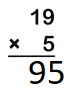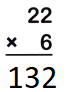# McGraw Hill Math Grade 4 Chapter 5 Lesson 1 Answer Key Multiplying a Two-Digit Number

Practice the questions of McGraw Hill Math Grade 4 Answer Key PDF Chapter 5 Lesson 1 Multiplying a Two-Digit Number to secure good marks & knowledge in the exams.

## McGraw-Hill Math Grade 4 Answer Key Chapter 5 Lesson 1 Multiplying a Two-Digit Number

Multiply

Find each product using partial products.

Question 1.13 x 4 = 52
Explanation:
The product of 13 and 4 is 52
take the carried value and place it in the column to the left.
So if you were multiplying numbers in the tens column and you needed to carry a number,
you would place that “number” in the hundreds column.

Question 2.Explanation:
The product of 19 and 5 is 95
take the carried value and place it in the column to the left.
So if you were multiplying numbers in the tens column and you needed to carry a number,
you would place that “number” in the hundreds column.

Question 3.Explanation:
The product of 22 and 6 is 132
take the carried value and place it in the column to the left.
So if you were multiplying numbers in the tens column and you needed to carry a number,
you would place that “number” in the hundreds column.

Question 4.Explanation:
The product of 90 and 6 is 540
take the carried value and place it in the column to the left.
So if you were multiplying numbers in the tens column and you needed to carry a number,
you would place that “number” in the hundreds column.

Question 5.
The owner of a restaurant buys 26 tables. She wants to buy 5 chairs for each table. How many chairs should she buy in all?